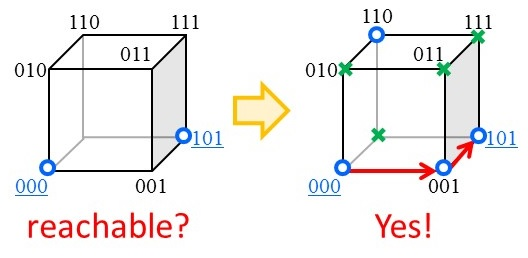# Web Portal for Combinatorial Reconfiguration

This is a website portal for Combinatorial Reconfiguration (CoRe).

### What is "combinatorial reconfiguration" ?

Reconfiguration problems arise when we wish to find a step-by-step transformation between feasible solutions of a combinatorial (search) problem such that all intermediate results are also feasible. For example, given two specified satisfying assignments A and B to a Boolean formula, one might ask whether A can be transformed into B by changing the assignment of one variable at a time such that each intermediate assignment is also satisfying. Thus, a reconfiguration problem asks the "reachability" on the solution space, whereas the original search problem asks the "existence" of at least one feasible solution.